# Methods and formulas for the model summary in CART® Classification

Select the method or formula of your choice.

## Important predictors

The number of predictors with positive relative importance.

Any classification tree is a collection of splits. Each split provides improvement to the tree. Each split also includes surrogate splits that also provide improvement to the tree. The importance of a variable is given by all of its improvements when the tree uses the variable to split a node or as a surrogate to split a node when another variable has a missing value.

The following formula gives the improvement at a single node:

The values of I(t), pLeft, and pRight depend on the criterion for splitting the nodes. For more information, go to Node splitting methods in CART® Classification.

The formula for the relative importance for the qth predictor scales the importance by the most important variable:

## Deviance R-squared

Minitab calculates the deviance R-squared value when the response is binary. The following equation gives the formula for deviance R2:

The calculation of the deviance values depends on the validation technique.

### Notation for training data or no validation

TermDescriptionprobability of the event in the data
Nsample size of the full data or of the training data
wiweight for the ith observation in the full data set or the training data set
yiindicator variable that is 1 for the event and 0 otherwise in the full data set or the training data setmean log-likelihood

### K-fold cross validation

For cross-validation, the calculations omit one fold at a time.

### Notation for k-fold cross-validation

TermDescription
Knumber of foldsprobability of the event in the data that does not include the observations in fold j
njsample size of fold j
wijweight for the ith observation in fold j
yijindicator variable that is 1 for the event and 0 otherwise for the data in fold jpredicted probability of the event from the model estimation that does not include the observations for the ith observation in fold j

### Test data set

The calculations for the test data set are similar to the calculations for the training data but use the test data.

### Notation for test data set

TermDescriptionprobability of the event in the training data set
nTestsample size of the test data set
wi, Testweight for the ith observation in the test data set
yi, Testindicator variable that is 1 for the event and 0 otherwise for data in the test data setmean log-likelihood

## Average –log-likelihood

Minitab calculates the average of the negative log-likelihood function when the response is binary. The calculations depend on the validation method.

where

### Notation for training data or no validation

TermDescription
Nsample size of the full data or the training data
wiweight for the ith observation in the full or training data set
yiindicator variable that is 1 for the event and 0 otherwise for the full or training data set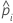predicted probability of the event for the ith row in the full or training data set

where

### Notation for k-fold cross-validation

TermDescription
Nsample size of the full or training data
njsample size of fold j
wijweight for the ith observation in fold j
yijindicator variable that is 1 for the event and 0 otherwise for the data in fold j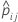predicted probability of the event from the model estimation that does not include the observations for the ith observation in fold j

where

### Notation for test data set

TermDescription
nTestsample size of the test data set
wi, Testweight for the ith observation in the test data set
yi, Testindicator variable that is 1 for the event and 0 otherwise for the data in the test data setpredicted probability of the event for the ith row in the training data set

## Area under ROC curve

The ROC curve plots the true positive rate (TPR), also known as power, on the y-axis, and the false positive rate (FPR), also known as type 1 error, on the x-axis. The area under the ROC curve values range from 0.5 to 1.

### Formula

For the area under the curve, Minitab uses an integration.

In most cases, this integral is equivalent to the following summation of areas of trapezoids:

where k is the number of terminal nodes and (x0, y0) is the point (0, 0).

For example, suppose your results have 4 terminal nodes with the following coordinates on the ROC curve:
x (false positive rate) y (true positive rate)
0.0923 0.3051
0.4154 0.7288
0.7538 0.9322
1 1
Then the area under the ROC curve is given by the following calculation: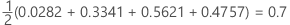### Notation

TermDescription
TRP true positive rate
FPR false positive rate
TPtrue positive, events that were correctly assessed
P number of actual positive events
FPtrue negative, nonevents that were correctly assessed
N number of actual negative events
FNRfalse negative rate
TNRtrue negative rate

## 95% CI for the area under the ROC curve

Minitab calculates a confidence interval for the area under the Receiver Operating Characteristic curve when the response is binary.

The following interval gives the upper and lower bounds for the confidence interval:

The computation of the standard error of the area under the ROC curve (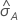) comes from Salford Predictive Modeler®. For general information about estimation of the variance of the area under the ROC curve, see the following references:

Engelmann, B. (2011). Measures of a ratings discriminative power: Applications and limitations. In B. Engelmann & R. Rauhmeier (Eds.), The Basel II Risk Parameters: Estimation, Validation, Stress Testing - With Applications to Loan Risk Management (2nd ed.) Heidelberg; New York: Springer. doi:10.1007/978-3-642-16114-8

Cortes, C. and Mohri, M. (2005). Confidence intervals for the area under the ROC curve. Advances in neural information processing systems, 305-312.

Feng, D., Cortese, G., & Baumgartner, R. (2017). A comparison of confidence/credible interval methods for the area under the ROC curve for continuous diagnostic tests with small sample size. Statistical Methods in Medical Research, 26(6), 2603-2621. doi:10.1177/0962280215602040

### Notation

TermDescription
Aarea under the ROC curve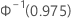percentile of the standard normal distribution

## Lift

Minitab displays lift in the model summary table when the response is binary. The lift in the model summary table is the cumulative lift for the 10% of the data with the best chance of correct classification.

### Formula

For the 10% of observations in the data with the highest probabilities of being assigned to the event class, use the following formula.

For the test lift with a test data set, use observations from the test data set. For the test lift with k-fold cross-validation, select the data to use and calculate the lift from the predicted probabilities for data that are not in the model estimation.

### Notation

TermDescription
dnumber of cases in 10% of the data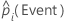predicted probability of the event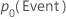probability of the event in the training data or, if the analysis uses no validation, in the full data set

## Misclassification cost

The misclassification cost in the model summary table is the relative misclassification cost for the model relative to a trivial classifier that classifies all observations into the most frequent class.

To find the misclassification cost, begin with the following definition:

The relative misclassification cost has the following form:

Where R0 is the cost for the trivial classifier.

The formula for R simplifies when the prior probabilities are equal or are from the data.

### Equal prior probabilities

When the prior probabilities are equal, the following definition applies:
With this definition, R has the following form:

### Prior probabilities from the data

When the prior probabilities are from the data, the following definition applies:

With this definition, R has the following form:

### Notation

TermDescription
πjprior probability of the jth class of the response variable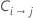cost of misclassifying class i as class j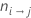number of class i records misclassified as class j
Njnumber of cases in the jth class of the response variable
Knumber of classes in the response variable
Nnumber of cases in the data
By using this site you agree to the use of cookies for analytics and personalized content.  Read our policy